Скачать презентацию The Gaseous State of Matter Preparation for College

e3fb37c43b3610dc2ba4379925c92007.ppt

• Количество слайдов: 34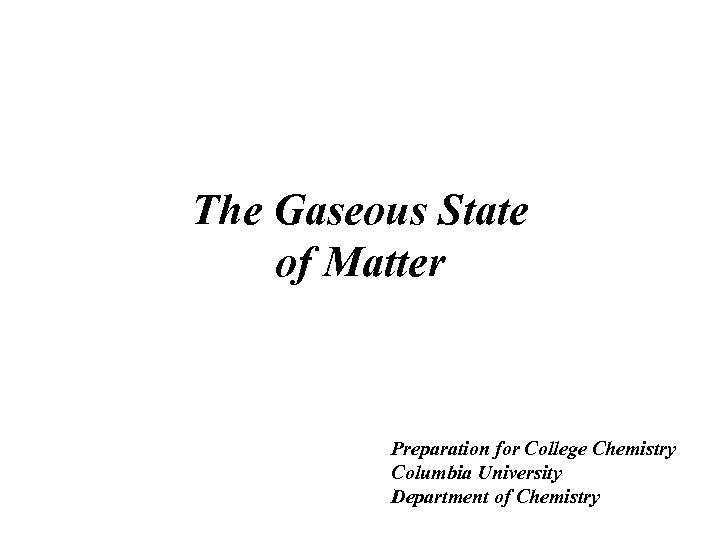The Gaseous State of Matter Preparation for College Chemistry Columbia University Department of Chemistry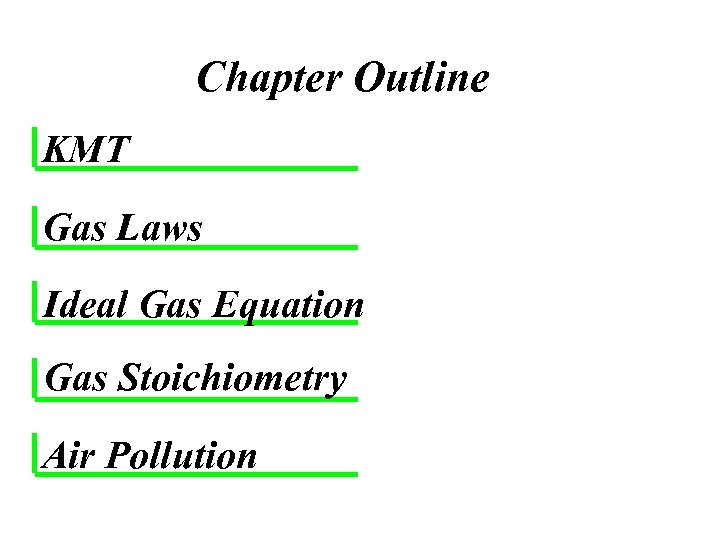Chapter Outline KMT Gas Laws Ideal Gas Equation Gas Stoichiometry Air Pollution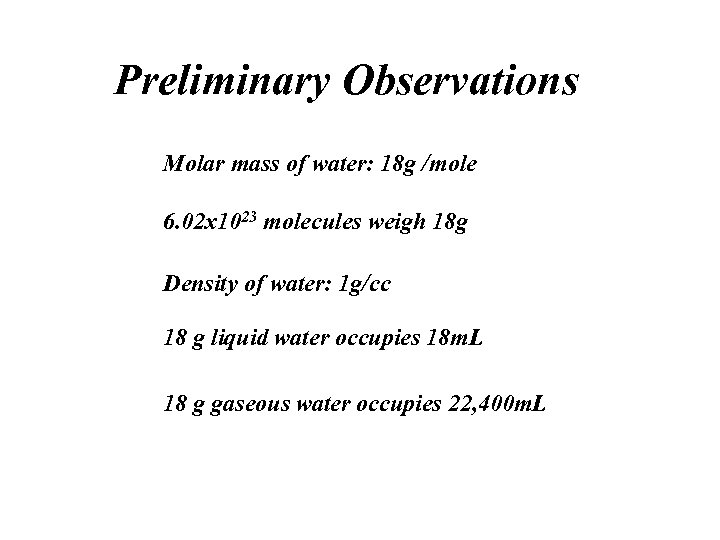Preliminary Observations Molar mass of water: 18 g /mole 6. 02 x 1023 molecules weigh 18 g Density of water: 1 g/cc 18 g liquid water occupies 18 m. L 18 g gaseous water occupies 22, 400 m. L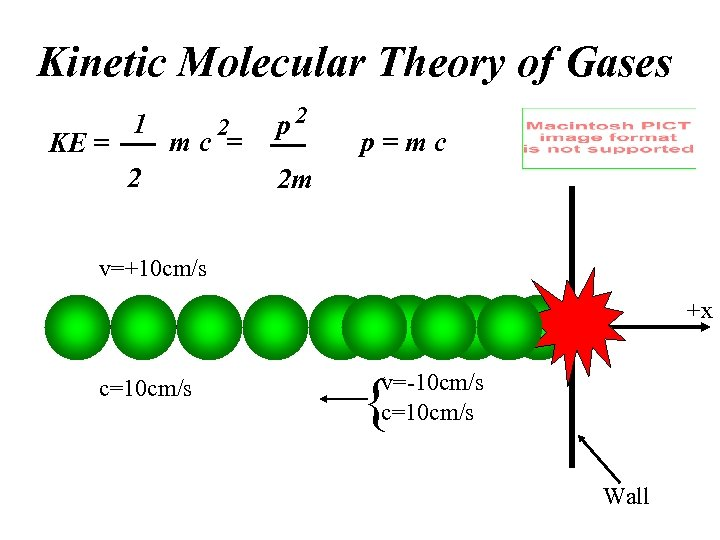Kinetic Molecular Theory of Gases KE = 1 2 mc = 2 p=mc 2 m v=+10 cm/s -x c=10 cm/s +x { v=-10 cm/s c=10 cm/s Wall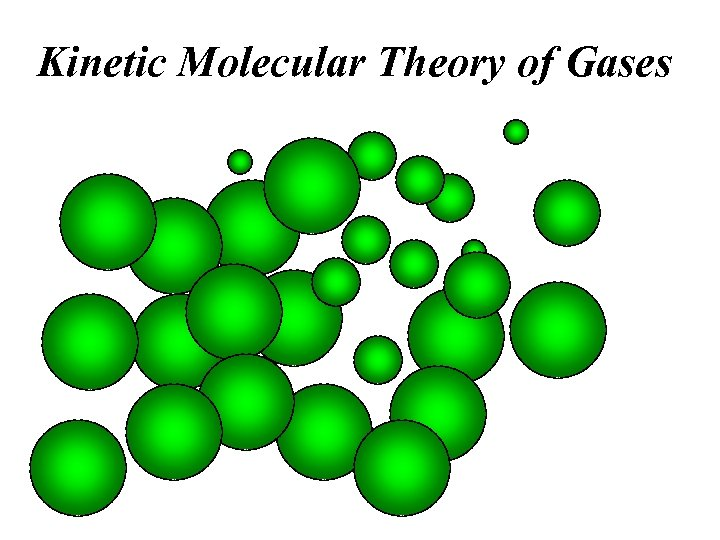Kinetic Molecular Theory of Gases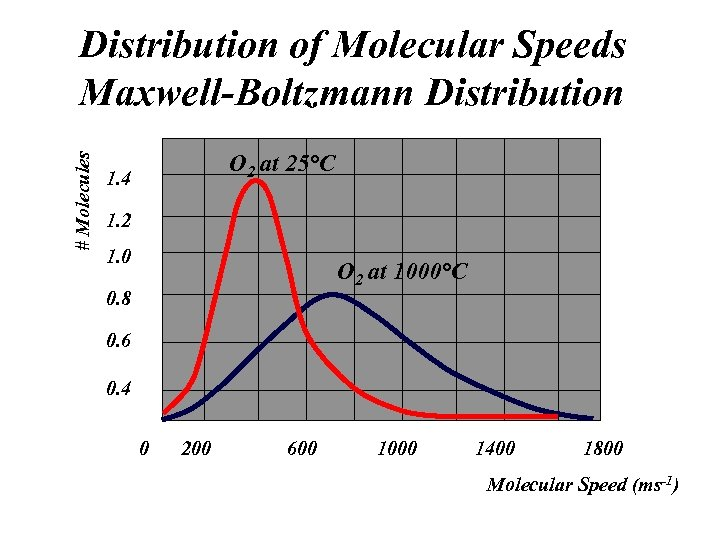# Molecules Distribution of Molecular Speeds Maxwell-Boltzmann Distribution O 2 at 25°C 1. 4 1. 2 1. 0 O 2 at 1000°C 0. 8 0. 6 0. 4 0 200 600 1000 1400 1800 Molecular Speed (ms-1)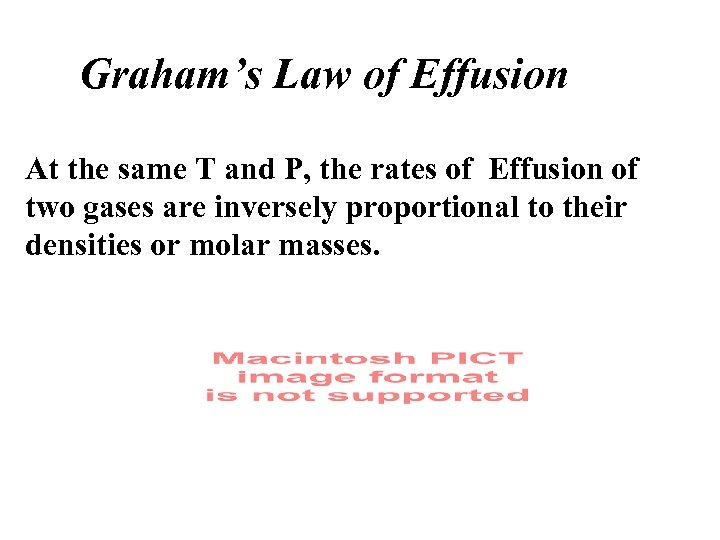Graham’s Law of Effusion At the same T and P, the rates of Effusion of two gases are inversely proportional to their densities or molar masses.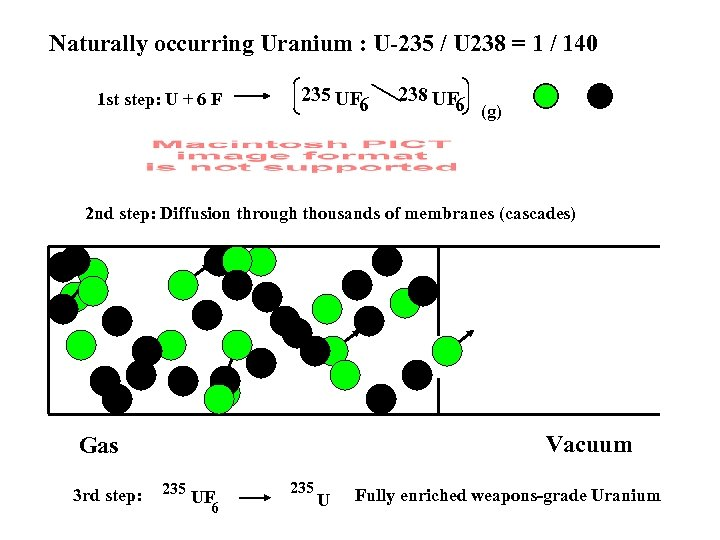Naturally occurring Uranium : U-235 / U 238 = 1 / 140 1 st step: U + 6 F 235 UF 6 238 UF 6 (g) 2 nd step: Diffusion through thousands of membranes (cascades) Vacuum Gas 3 rd step: 235 UF 6 235 U Fully enriched weapons-grade Uranium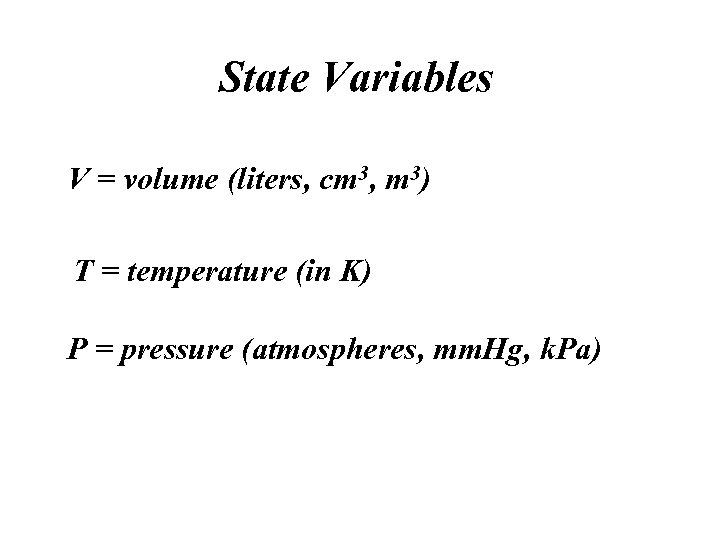State Variables V = volume (liters, cm 3, m 3) T = temperature (in K) P = pressure (atmospheres, mm. Hg, k. Pa)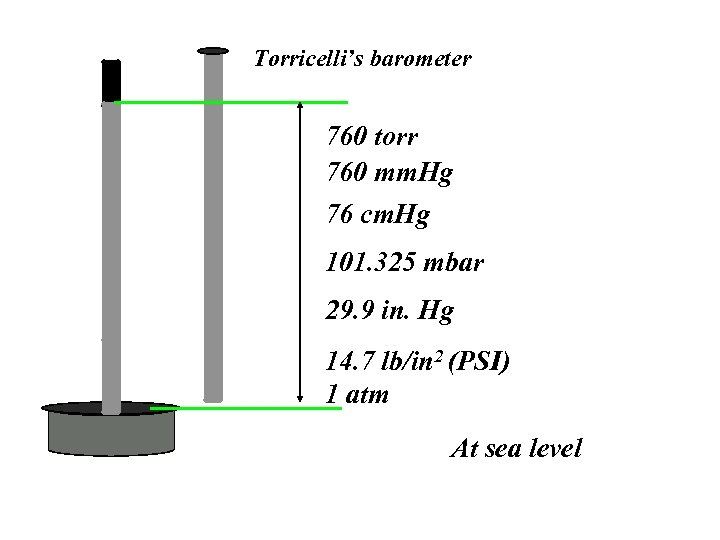Torricelli’s barometer 760 torr 760 mm. Hg 76 cm. Hg 101. 325 mbar 29. 9 in. Hg 14. 7 lb/in 2 (PSI) 1 atm At sea level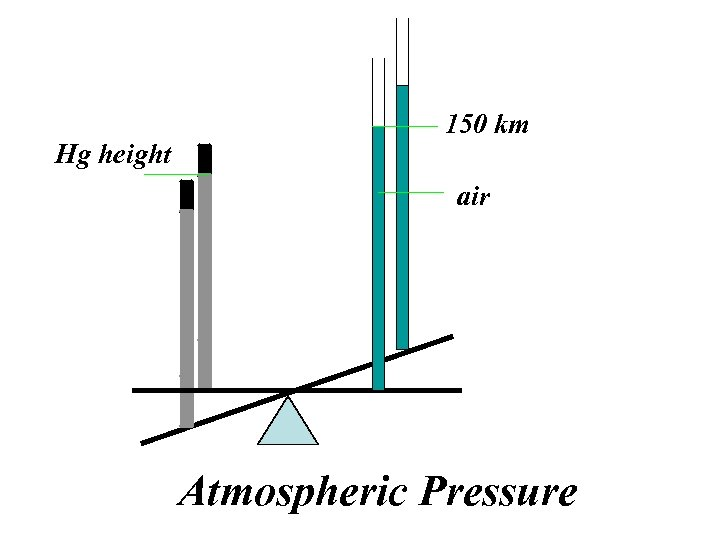Hg height 150 km air Atmospheric Pressure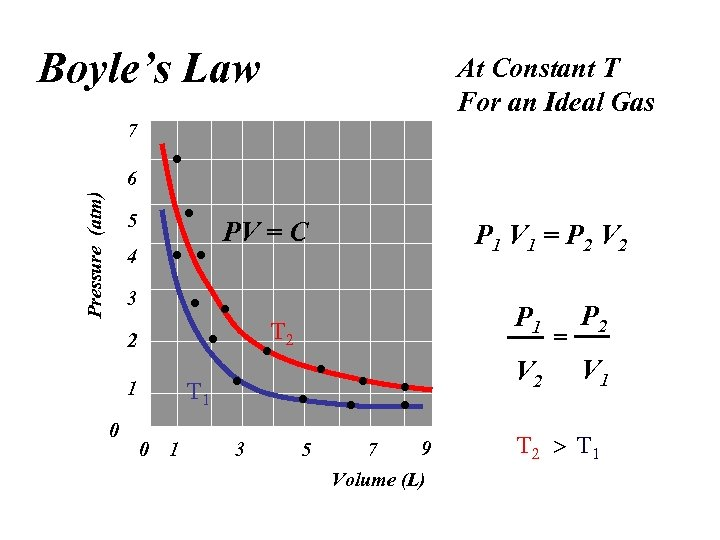Boyle’s Law At Constant T For an Ideal Gas 7 Pressure (atm) 6 5 PV = C 4 P 1 V 1 = P 2 V 2 3 2 V 2 1 0 P 1 0 1 3 5 9 7 Volume (L) = P 2 V 1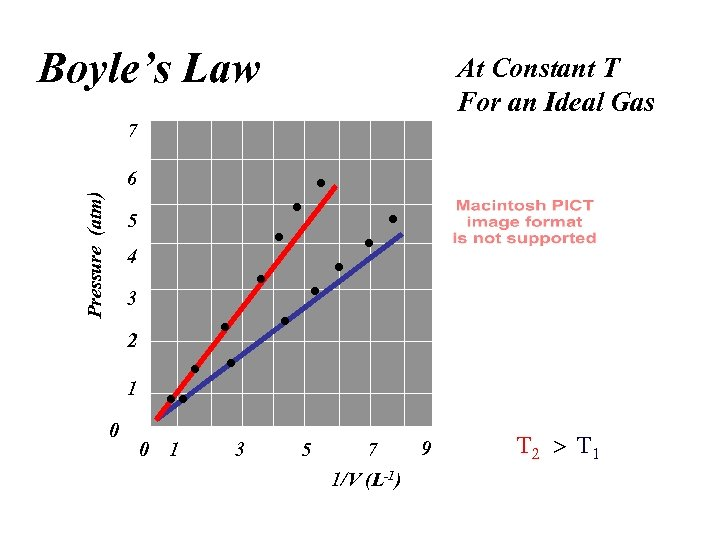Boyle’s Law At Constant T For an Ideal Gas 7 Pressure (atm) 6 5 4 3 2 1 0 0 1 3 5 9 7 1/V (L-1)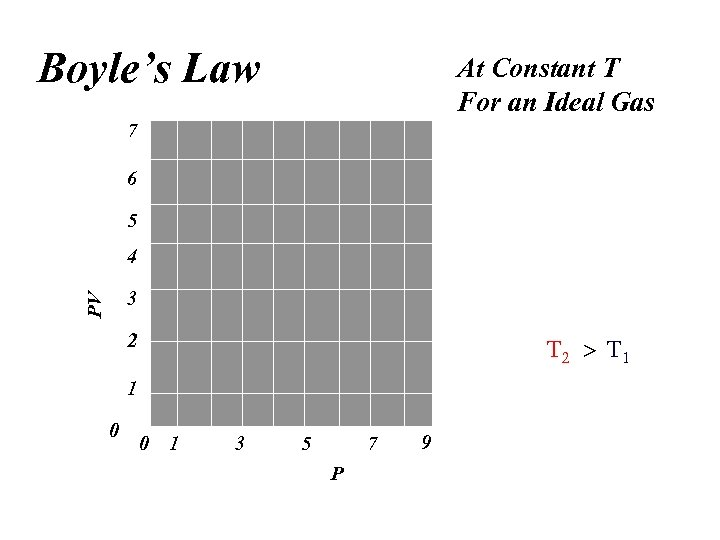Boyle’s Law At Constant T For an Ideal Gas 7 6 5 4 PV 3 2 1 0 0 1 3 5 7 P 9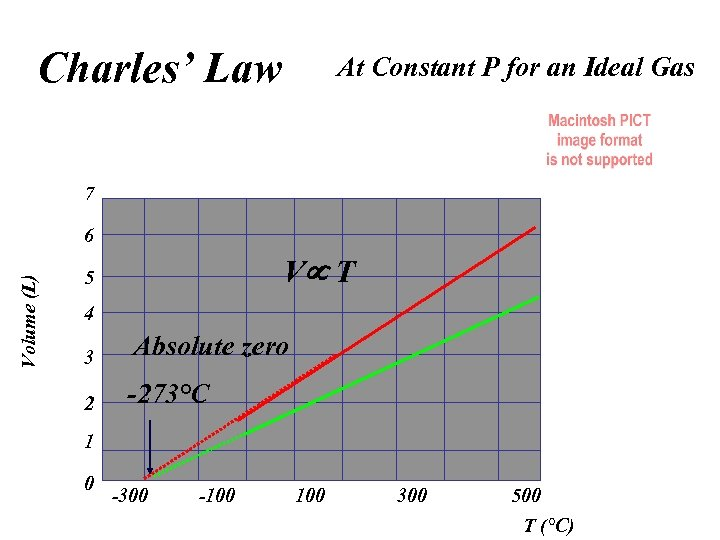Charles’ Law At Constant P for an Ideal Gas 7 Volume (L) 6 V T 5 4 3 2 Absolute zero -273°C 1 0 -300 -100 300 500 T (°C)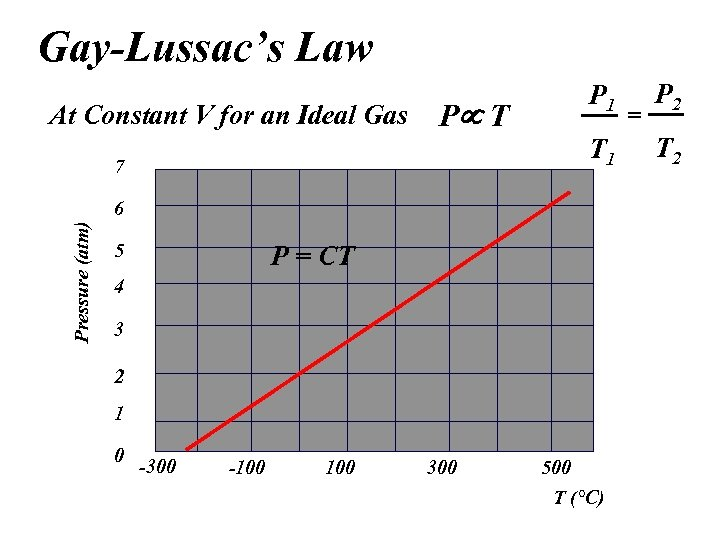Gay-Lussac’s Law At Constant V for an Ideal Gas P 1 P T T 1 7 Pressure (atm) 6 5 P = CT 4 3 2 1 0 -300 -100 300 500 T (°C) = P 2 T 2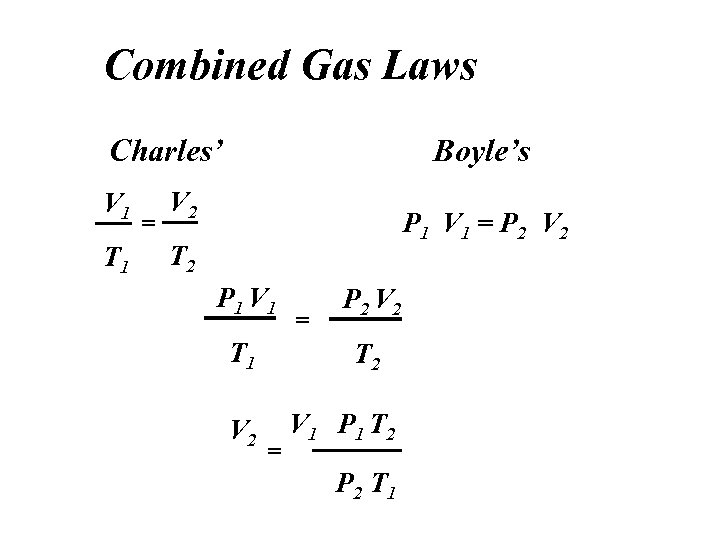Combined Gas Laws Charles’ V 1 T 1 = Boyle’s V 2 P 1 V 1 = P 2 V 2 T 2 P 1 V 1 T 1 V 2 = P 2 V 2 T 2 = V 1 P 1 T 2 P 2 T 1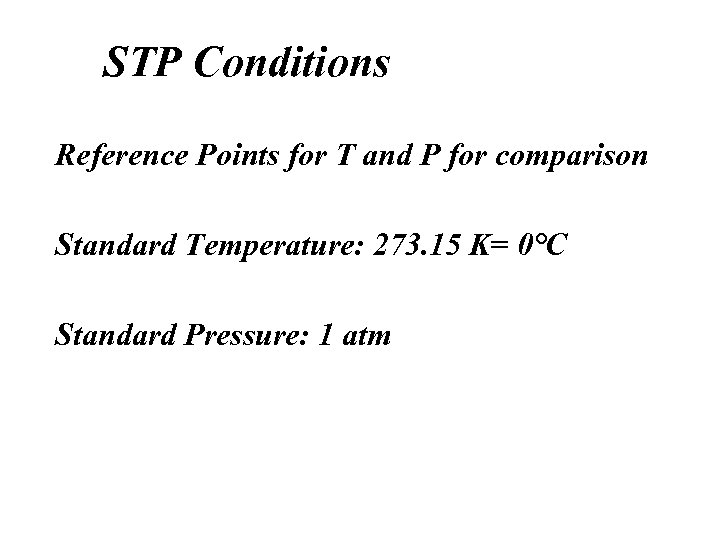STP Conditions Reference Points for T and P for comparison Standard Temperature: 273. 15 K= 0°C Standard Pressure: 1 atm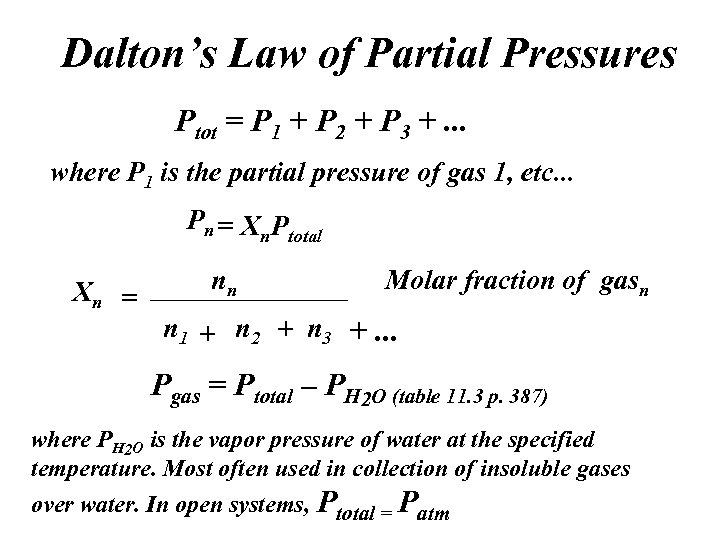Dalton’s Law of Partial Pressures Ptot = P 1 + P 2 + P 3 +. . . where P 1 is the partial pressure of gas 1, etc. . . Pn = Xn Ptotal Xn = nn Molar fraction of gasn n 1 + n 2 + n 3 +. . . Pgas = Ptotal – PH 2 O (table 11. 3 p. 387) where PH 2 O is the vapor pressure of water at the specified temperature. Most often used in collection of insoluble gases over water. In open systems, Ptotal = Patm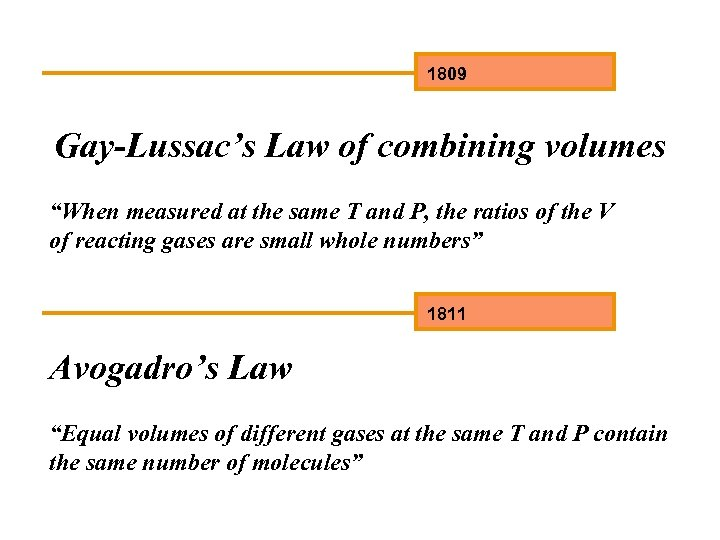1809 Gay-Lussac’s Law of combining volumes “When measured at the same T and P, the ratios of the V of reacting gases are small whole numbers” 1811 Avogadro’s Law “Equal volumes of different gases at the same T and P contain the same number of molecules”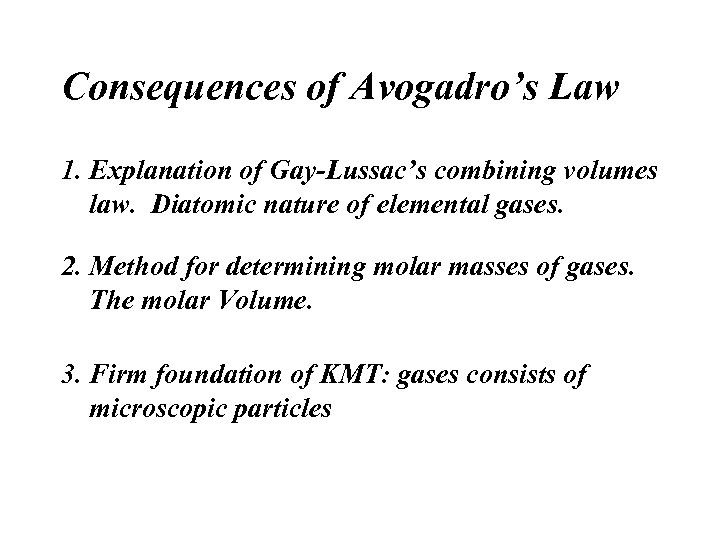Consequences of Avogadro’s Law 1. Explanation of Gay-Lussac’s combining volumes law. Diatomic nature of elemental gases. 2. Method for determining molar masses of gases. The molar Volume. 3. Firm foundation of KMT: gases consists of microscopic particles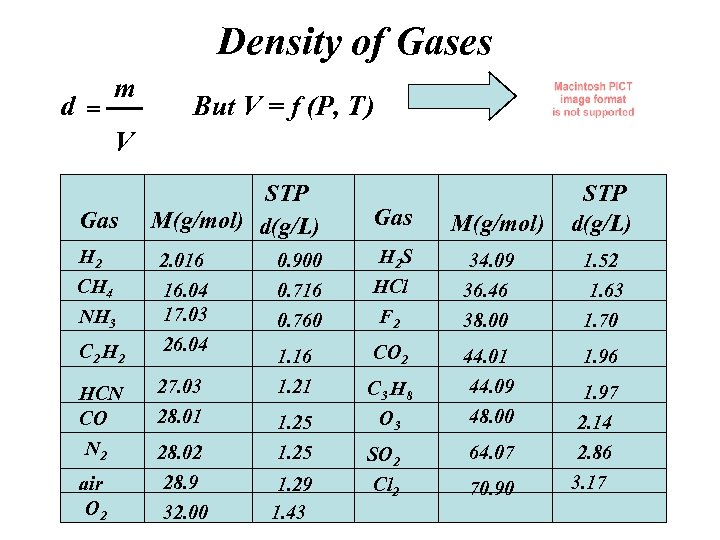Density of Gases d= m But V = f (P, T) V Gas H 2 CH 4 NH 3 C 2 H 2 STP M(g/mol) d(g/L) 2. 016 16. 04 17. 03 26. 04 0. 900 0. 716 0. 760 1. 16 1. 21 HCN CO 27. 03 28. 01 N 2 28. 02 1. 25 air O 2 28. 9 32. 00 1. 29 1. 43 Gas H 2 S HCl F 2 CO 2 C 3 H 8 O 3 SO 2 Cl 2 M(g/mol) STP d(g/L) 34. 09 36. 46 38. 00 1. 52 1. 63 1. 70 44. 01 44. 09 48. 00 1. 96 1. 97 64. 07 2. 14 2. 86 70. 90 3. 17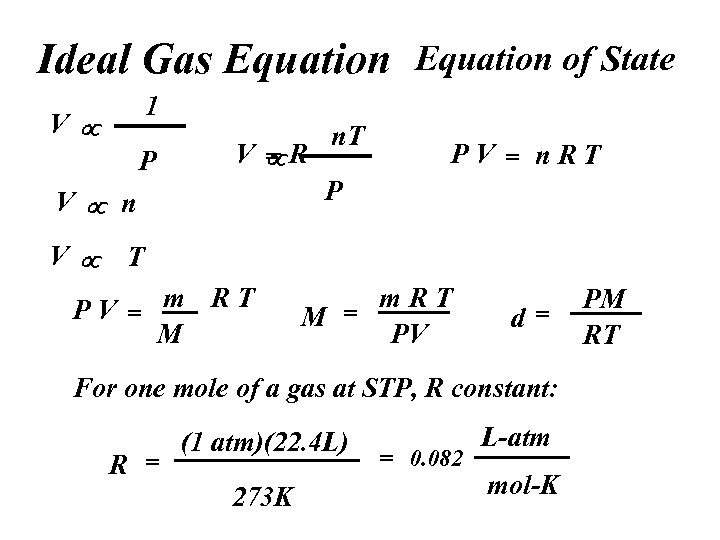Ideal Gas Equation of State 1 V P V R = PV = n. RT P V n. T T PV = m RT M m. RT M = PV d= For one mole of a gas at STP, R constant: R = (1 atm)(22. 4 L) 273 K = 0. 082 L-atm mol-K PM RT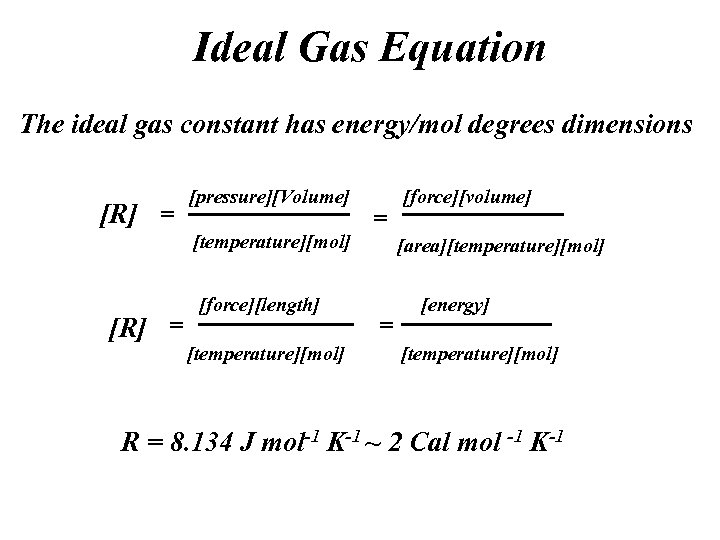Ideal Gas Equation The ideal gas constant has energy/mol degrees dimensions [R] = [pressure][Volume] [temperature][mol] [R] = [force][length] [temperature][mol] = [force][volume] [area][temperature][mol] = [energy] [temperature][mol] R = 8. 134 J mol-1 K-1 ~ 2 Cal mol -1 K-1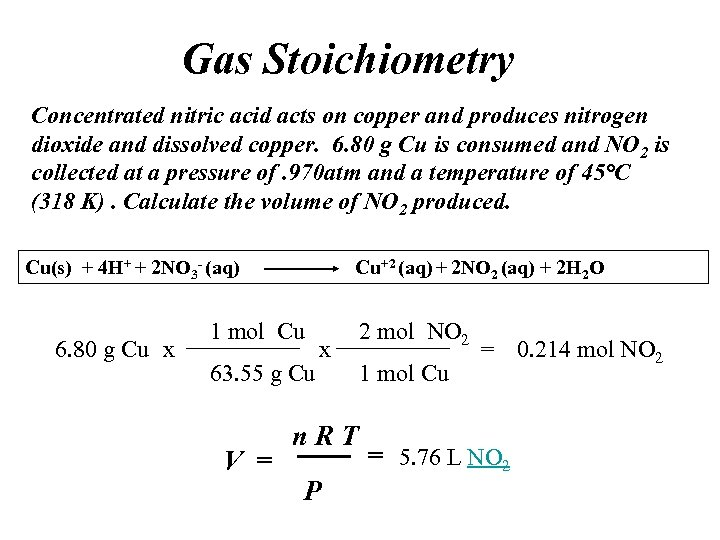Gas Stoichiometry Concentrated nitric acid acts on copper and produces nitrogen dioxide and dissolved copper. 6. 80 g Cu is consumed and NO 2 is collected at a pressure of. 970 atm and a temperature of 45°C (318 K). Calculate the volume of NO 2 produced. Cu(s) + 4 H+ + 2 NO 3 - (aq) 6. 80 g Cu x Cu+2 (aq) + 2 NO 2 (aq) + 2 H 2 O 1 mol Cu 63. 55 g Cu V = x n. RT P 2 mol NO 2 1 mol Cu = 0. 214 mol NO 2 = 5. 76 L NO 2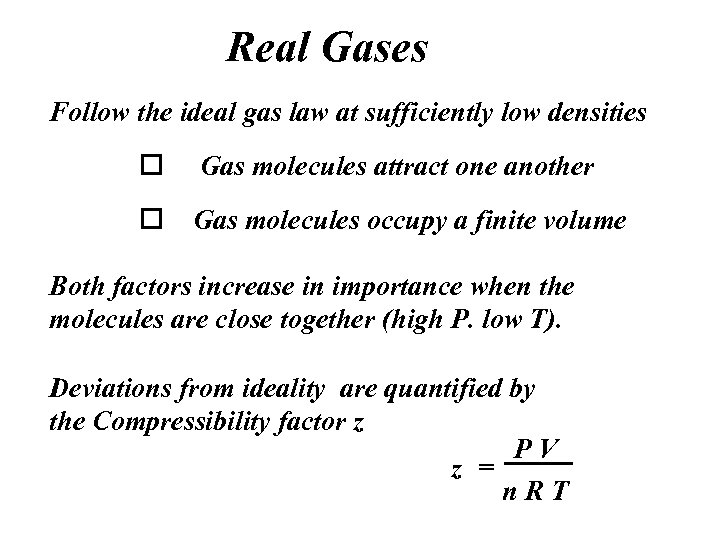Real Gases Follow the ideal gas law at sufficiently low densities o Gas molecules attract one another o Gas molecules occupy a finite volume Both factors increase in importance when the molecules are close together (high P. low T). Deviations from ideality are quantified by the Compressibility factor z PV z = n. RT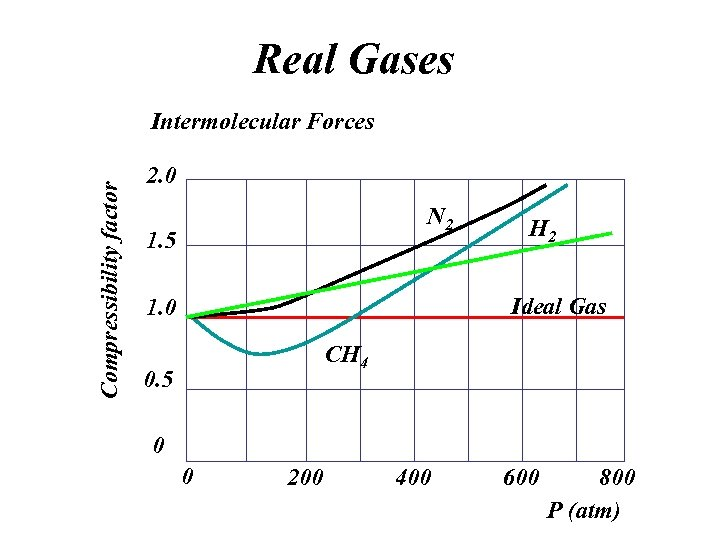Real Gases Compressibility factor Intermolecular Forces 2. 0 N 2 1. 5 H 2 Ideal Gas 1. 0 CH 4 0. 5 0 0 200 400 600 800 P (atm)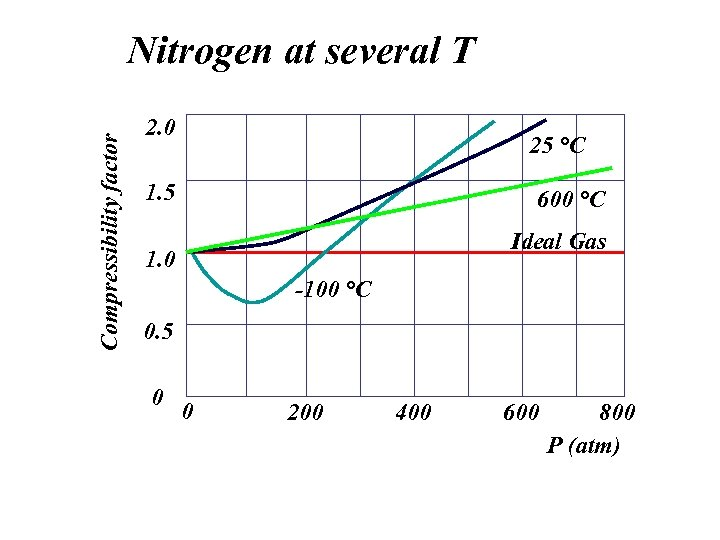Compressibility factor Nitrogen at several T 2. 0 25 °C 1. 5 600 °C Ideal Gas 1. 0 -100 °C 0. 5 0 0 200 400 600 800 P (atm)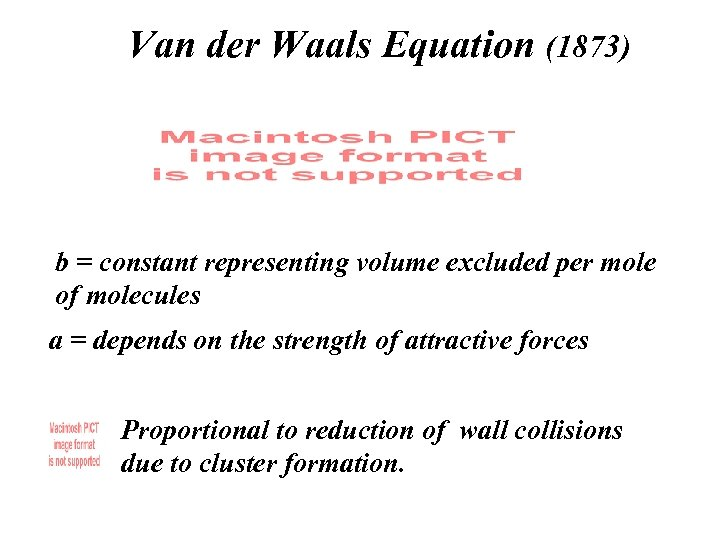Van der Waals Equation (1873) b = constant representing volume excluded per mole of molecules a = depends on the strength of attractive forces Proportional to reduction of wall collisions due to cluster formation.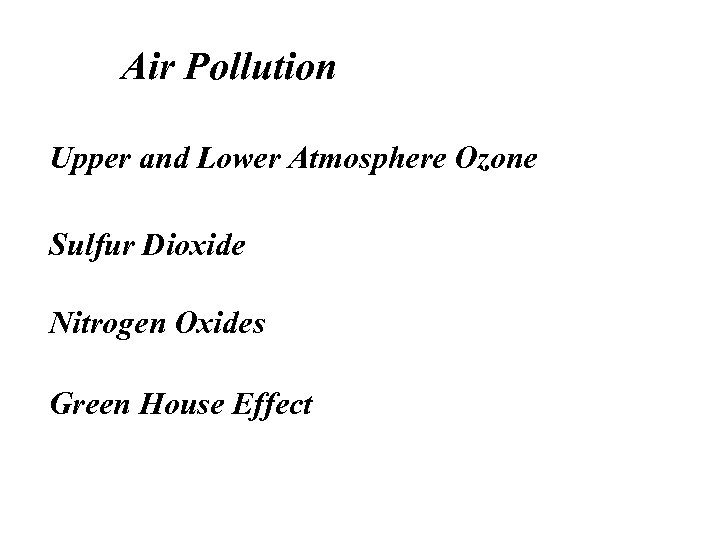Air Pollution Upper and Lower Atmosphere Ozone Sulfur Dioxide Nitrogen Oxides Green House Effect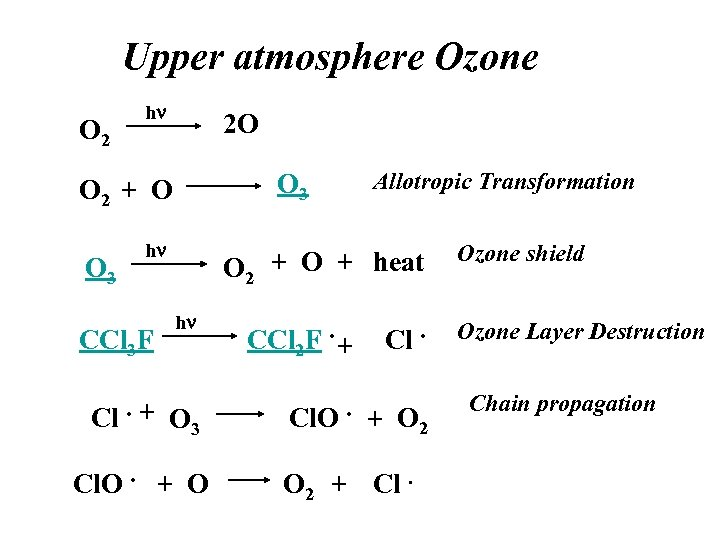Upper atmosphere Ozone O 2 hn 2 O O 3 O 2 + O O 3 hn CCl 3 F Cl. + Allotropic Transformation O 2 + O + heat hn CCl 2 F. + O 3 Cl. O. + O Cl. + O 2 + Cl. Ozone shield Ozone Layer Destruction Chain propagation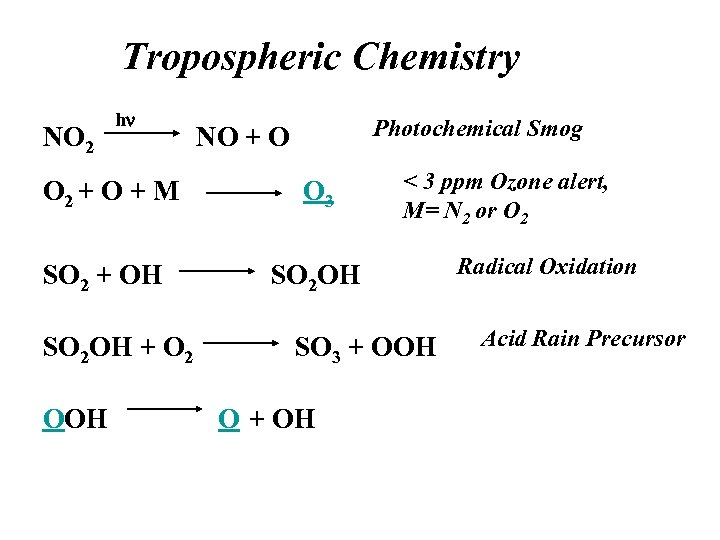Tropospheric Chemistry NO 2 hn Photochemical Smog NO + O O 2 + O + M O 3 SO 2 + OH < 3 ppm Ozone alert, M= N 2 or O 2 SO 2 OH + O 2 OOH SO 3 + OOH O + OH Radical Oxidation Acid Rain Precursor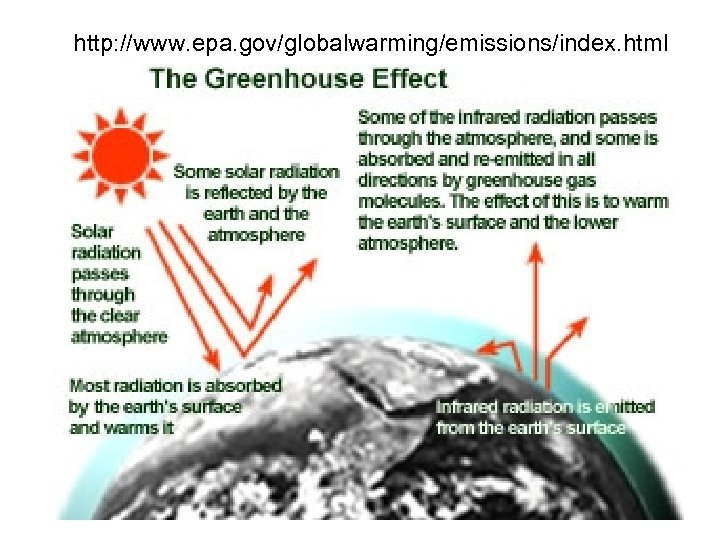http: //www. epa. gov/globalwarming/emissions/index. html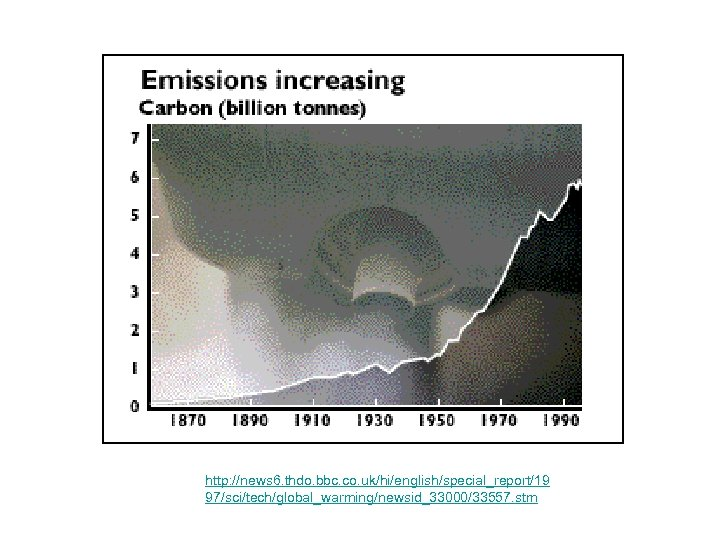http: //news 6. thdo. bbc. co. uk/hi/english/special_report/19 97/sci/tech/global_warming/newsid_33000/33557. stm